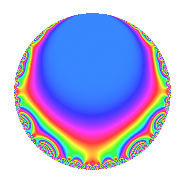# Properties

 Label 418.2.b.aLevel 418 Weight 2 Character orbit 418.b Analytic conductor 3.338 Analytic rank 0 Dimension 2 CM No Inner twists 2

# Related objects

## Newspace parameters

 Level: $$N$$ = $$418 = 2 \cdot 11 \cdot 19$$ Weight: $$k$$ = $$2$$ Character orbit: $$[\chi]$$ = 418.b (of order $$2$$ and degree $$1$$)

## Newform invariants

 Self dual: No Analytic conductor: $$3.33774680449$$ Analytic rank: $$0$$ Dimension: $$2$$ Coefficient field: $$\Q(\sqrt{-10})$$ Coefficient ring: $$\Z[a_1, \ldots, a_{7}]$$ Coefficient ring index: $$1$$ Sato-Tate group: $\mathrm{SU}(2)[C_{2}]$

## $q$-expansion

Coefficients of the $$q$$-expansion are expressed in terms of $$\beta = \sqrt{-10}$$. We also show the integral $$q$$-expansion of the trace form.

 $$f(q)$$ $$=$$ $$q - q^{2} + q^{4} -2 q^{5} + \beta q^{7} - q^{8} + 3 q^{9} +O(q^{10})$$ $$q - q^{2} + q^{4} -2 q^{5} + \beta q^{7} - q^{8} + 3 q^{9} + 2 q^{10} + ( 1 - \beta ) q^{11} -6 q^{13} -\beta q^{14} + q^{16} + 2 \beta q^{17} -3 q^{18} + ( -3 + \beta ) q^{19} -2 q^{20} + ( -1 + \beta ) q^{22} -4 q^{23} - q^{25} + 6 q^{26} + \beta q^{28} + 6 q^{29} + 3 \beta q^{31} - q^{32} -2 \beta q^{34} -2 \beta q^{35} + 3 q^{36} + 3 \beta q^{37} + ( 3 - \beta ) q^{38} + 2 q^{40} + ( 1 - \beta ) q^{44} -6 q^{45} + 4 q^{46} -8 q^{47} -3 q^{49} + q^{50} -6 q^{52} -3 \beta q^{53} + ( -2 + 2 \beta ) q^{55} -\beta q^{56} -6 q^{58} -\beta q^{61} -3 \beta q^{62} + 3 \beta q^{63} + q^{64} + 12 q^{65} + 2 \beta q^{68} + 2 \beta q^{70} + 3 \beta q^{71} -3 q^{72} -3 \beta q^{74} + ( -3 + \beta ) q^{76} + ( 10 + \beta ) q^{77} -2 q^{80} + 9 q^{81} -2 \beta q^{83} -4 \beta q^{85} + ( -1 + \beta ) q^{88} + 6 q^{90} -6 \beta q^{91} -4 q^{92} + 8 q^{94} + ( 6 - 2 \beta ) q^{95} -6 \beta q^{97} + 3 q^{98} + ( 3 - 3 \beta ) q^{99} +O(q^{100})$$ $$\operatorname{Tr}(f)(q)$$ $$=$$ $$2q - 2q^{2} + 2q^{4} - 4q^{5} - 2q^{8} + 6q^{9} + O(q^{10})$$ $$2q - 2q^{2} + 2q^{4} - 4q^{5} - 2q^{8} + 6q^{9} + 4q^{10} + 2q^{11} - 12q^{13} + 2q^{16} - 6q^{18} - 6q^{19} - 4q^{20} - 2q^{22} - 8q^{23} - 2q^{25} + 12q^{26} + 12q^{29} - 2q^{32} + 6q^{36} + 6q^{38} + 4q^{40} + 2q^{44} - 12q^{45} + 8q^{46} - 16q^{47} - 6q^{49} + 2q^{50} - 12q^{52} - 4q^{55} - 12q^{58} + 2q^{64} + 24q^{65} - 6q^{72} - 6q^{76} + 20q^{77} - 4q^{80} + 18q^{81} - 2q^{88} + 12q^{90} - 8q^{92} + 16q^{94} + 12q^{95} + 6q^{98} + 6q^{99} + O(q^{100})$$

## Character Values

We give the values of $$\chi$$ on generators for $$\left(\mathbb{Z}/418\mathbb{Z}\right)^\times$$.

 $$n$$ $$287$$ $$343$$ $$\chi(n)$$ $$-1$$ $$-1$$

## Embeddings

For each embedding $$\iota_m$$ of the coefficient field, the values $$\iota_m(a_n)$$ are shown below.

For more information on an embedded modular form you can click on its label.

Label $$\iota_m(\nu)$$ $$a_{2}$$ $$a_{3}$$ $$a_{4}$$ $$a_{5}$$ $$a_{6}$$ $$a_{7}$$ $$a_{8}$$ $$a_{9}$$ $$a_{10}$$
417.1
 − 3.16228i 3.16228i
−1.00000 0 1.00000 −2.00000 0 3.16228i −1.00000 3.00000 2.00000
417.2 −1.00000 0 1.00000 −2.00000 0 3.16228i −1.00000 3.00000 2.00000
 $$n$$: e.g. 2-40 or 990-1000 Significant digits: Format: Complex embeddings Normalized embeddings Satake parameters Satake angles

## Inner twists

Char. orbit Parity Mult. Self Twist Proved
1.a Even 1 trivial yes
209.d Even 1 yes

## Hecke kernels

This newform can be constructed as the intersection of the kernels of the following linear operators acting on $$S_{2}^{\mathrm{new}}(418, [\chi])$$:

 $$T_{3}$$ $$T_{13} + 6$$